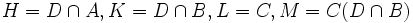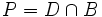# Zassenhaus isomorphism theorem

This article describes a fact or result that is not basic but it still well-established and standard. The fact may involve terms that are themselves non-basic
View other semi-basic facts in group theory
VIEW FACTS USING THIS: directly | directly or indirectly, upto two steps | directly or indirectly, upto three steps|
View a complete list of isomorphism theorems| Read a survey article about the isomorphism theorems

## Statement

Suppose$A, B, C, D$ are subgroups of a group$G$ such that$A$ is a normal subgroup of$B$ and$C$ is a normal subgroup of$D$. Then,$C(D \cap A)$ is normal in$C(D \cap B)$ and$A(B \cap C)$ is normal in$A(B \cap D)$, and we have an isomorphism:$\frac{C(D \cap B)}{C(D \cap A)} \cong \frac{A(B \cap D)}{A(B \cap C)}$.

## Facts used

1. Normality satisfies transfer condition: If$H$ is normal in$L$ and$K$ is a subgroup of$L$,$H \cap K$ is normal in$K$.
2. Modular property of groups: If$L \le M$, then$L(M \cap N) = M \cap LN$ and$(M \cap N)L = M \cap NL$.
3. Join lemma for normal subgroup of subgroup with normal subgroup of whole group: If$H \triangleleft K \le M$ and$L$ is normal in$M$, then the subgroup$\langle H, L \rangle = HL$ is normal in the subgroup$\langle K, L \rangle = KL$.
4. Second isomorphism theorem: If$P, Q$ are subgroups of a group$G$ such that$Q$ is normal in$PQ$, then$PQ/Q$ is isomorphic to$P/(P \cap Q)$.

## Proof

### Hands-on proof

Given:$A, B, C, D$ are subgroups of a group$G$ such that$A$ is a normal subgroup of$B$ and$C$ is a normal subgroup of$D$.

To prove:$C(D \cap A)$ is normal in$C(D \cap B)$ and$A(C \cap D) is normal in [itex]A(B \cap D)$, and we have an isomorphism:$\frac{C(D \cap B)}{C(D \cap A)} \cong \frac{A(B \cap D)}{A(B \cap C)}$.

Proof:

1. (Given data used:$A \triangleleft B$; Fact used: fact (1)):$D \cap A$ is normal in$D \cap B$: This follows from fact (1), setting$H = A, L = B, K = D \cap B$.
2. (Given data used:$C \triangleleft D$):$D \cap B$ and$D \cap A$ normalize$C$: Since$C$ is normal in$D$, the normalizer of$C$ contains$D$, hence it also contains$D \cap B$.
3.$C(D \cap B)$ and$C(D \cap A)$ are subgroups: This follows directly from step (2).
4.$C(D \cap A)$ is normal in$C(D \cap B)$: By step (2),$C$ is normal in$C(D \cap B)$, and by step (1),$D \cap A$ is normal in$D \cap B$. Thus, by fact (3), we obtain that$C(D \cap A)$ is normal in$C(D \cap B)$. (Here, we set$H = D \cap A, K = D \cap B, L = C, M = C(D \cap B)$).
5. (Facts used: fact (4)): Setting$P = D \cap B$ and$Q = C(D \cap A)$, we observe that by step (4),$Q$ is normal in$PQ$, so applying fact (4) yields:
•$\frac{C(D \cap B)}{C(D \cap A)} \cong \frac{D \cap B}{D \cap B \cap C(D \cap A)}$.
6. (Facts used: fact (2)): Applying fact (2) with$L = C, M = D, N = A$ yields$C(D \cap A) = D \cap CA = D \cap C$. (Note that both are equal because of the fact that$C$, being normal in$D$, permutes with$D \cap A$. We thus have:
•$\frac{C(D \cap B)}{C(D \cap A)} \cong \frac{D \cap B}{D \cap B \cap CA} = \frac{D \cap B}{D \cap B \cap AC}$.
7.$A (B \cap C)$ is normal in$A(B \cap D)$, both are subgroups, and we have (by analogous reasoning to steps (1) - (6)):
•$\frac{A(B \cap D)}{A(B \cap C)} \cong \frac{B \cap D}{B \cap D \cap AC} = \frac{B \cap D}{B \cap D \cap CA}$.
8. The right sides for steps (6) and (7) are equal, hence the left sides are isomorphic, completing the proof.